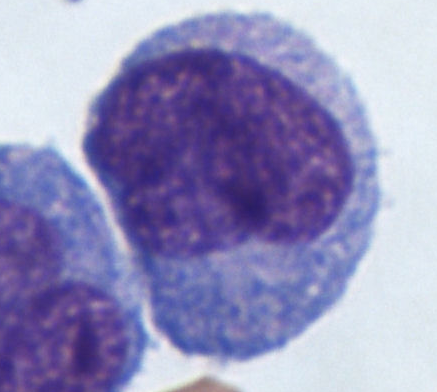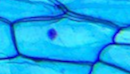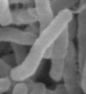GCSE Biology - AQA

1.1.5 - Magnification Calculations

# Magnification Calculations

Magnification, image size and actual size are related by the following equation:

Magnification = Image size ÷ Actual size

Note that image size means the size of the object in the image and actual size means the size of the actual object.

## Calculating the magnification of an image

Sometimes, you may need to calculate the magnification of an image. For example:

A cell is viewed through a microscope. The actual diameter of the cell is 80μm. In the image, the cell has a diameter of 32mm.

What is the magnification of the image?Image adapted from 'Monocytes, a type of white blood cell (Giemsa stained)' by Dr Graham Beards on Wikimedia Commons (CC BY-SA 3.0)

Before we can calculate the magnification, we need both diameters to be in the same units, so we start by converting 32mm to μm:

32mm = 32000μm

Then we divide the image size by the actual size:

Magnification = 32000 ÷ 80 = 400

Therefore, the image has a magnification of 400X.

## Calculating image size

Sometimes, you know the magnification and the actual size of an object and you need to calculate the image size. For example:

A plant cell is viewed through a microscope with a magnification of 350X. The actual diameter of the cell's nucleus is 14µm.

What is the diameter of the cell's nucleus in the image? Give your answer in mm.Image adapted from 'Onion skin cells under a microscope' by Katekor1 on Wikimedia Commons (CC BY 4.0)

First, we need to rearrange the formula to make image size the subject:

Image size = Actual size x Magnification

Then we multiply the actual size by the magnification to get the image size:

Image size = 14µm x 350 = 4900µm

Finally, we need to convert to mm, since this is what the question asked for:

4900µm = 4.9mm

The diameter of the nucleus in the image is 4.9mm.

## Calculating actual size

Sometimes you know the magnification and the image size and you need to calculate the actual size. For example:

Some bacterial cells are viewed using an electron microscope with a magnification of 20,000X. In the image formed, one of the cells is 8cm long.

What is the actual length of this cell? Give your answer in µm.First, we rearrange the formula to make actual size the subject:

Actual size = Image size ÷ Magnification

Then, we divide the image size by the magnification to get the actual size:

Actual size = 8cm ÷ 20,000 = 0.0004cm

Finally, we convert from cm to µm:

0.0004cm = 4µm

The actual length of the bacterial cell is 4µm.

## Flashcards

Flashcards help you memorise information quickly. Copy each question onto its own flashcard and then write the answer on the other side. Testing yourself on these regularly will enable you to learn much more quickly than just reading and making notes.

1/1

What is the equation linking image size, actual size and magnification?